## shufflev2-yolov5

Perform a series of ablation experiments on yolov5 to make it lighter (smaller Flops, lower memory, and fewer parameters) and faster (add shuffle channel, yolov5 head for channel reduce. It can infer at least 10+ FPS On the Raspberry Pi 4B when input the frame with 320×320) and is easier to deploy (removing the Focus layer and four slice operations, reducing the model quantization accuracy to an acceptable range).

### Comparison of ablation experiment results

ID Model Input_size Flops Params Size（M） [email protected] [email protected]:0.95
001 yolo-faster 320×320 0.25G 0.35M 1.4 24.4 -
002 nanodet-m 320×320 0.72G 0.95M 1.8 - 20.6
003 shufflev2-yolov5 320×320 1.43G 1.62M 3.3 35.5 -
004 nanodet-m 416×416 1.2G 0.95M 1.8 - 23.5
005 shufflev2-yolov5 416×416 2.42G 1.62M 3.3 40.5 23.5
006 yolov4-tiny 416×416 5.62G 8.86M 33.7 40.2 21.7
007 yolov3-tiny 416×416 6.96G 6.06M 23.0 33.1 16.6

### Comparison on different platforms

Equipment Computing backend System Framework Input Speed{our} Speed{yolov5s}
Inter @i5-10210U window(x86) 640×640 torch-cpu 112ms 179ms
Nvidia @RTX 2080Ti Linux(x86) 640×640 torch-gpu 11ms 13ms
Raspberrypi 4B @ARM Cortex-A72 Linux(arm64) 320×320 ncnn 97ms 371ms

### Detection effect

Pytorch{640×640}：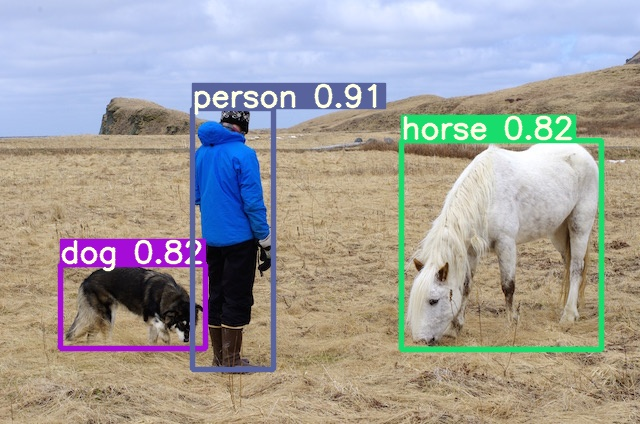NCNN{FP16}@{640×640}: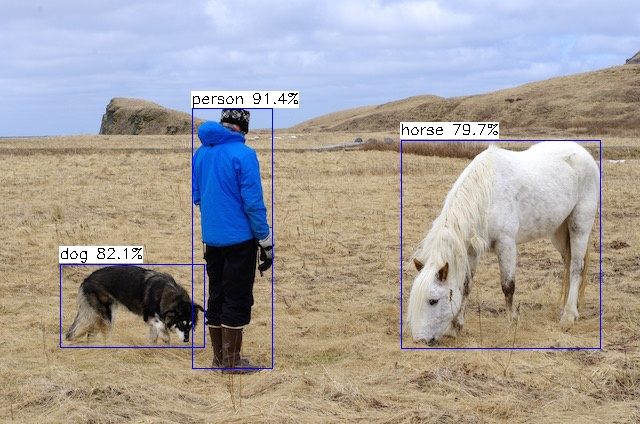NCNN{Int8}@{640×640}: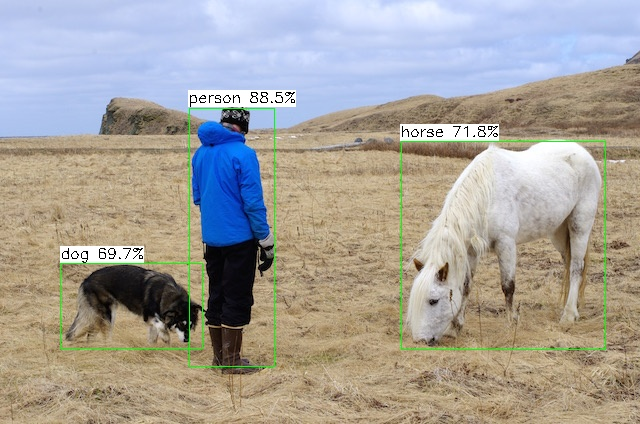### Base on YOLOv5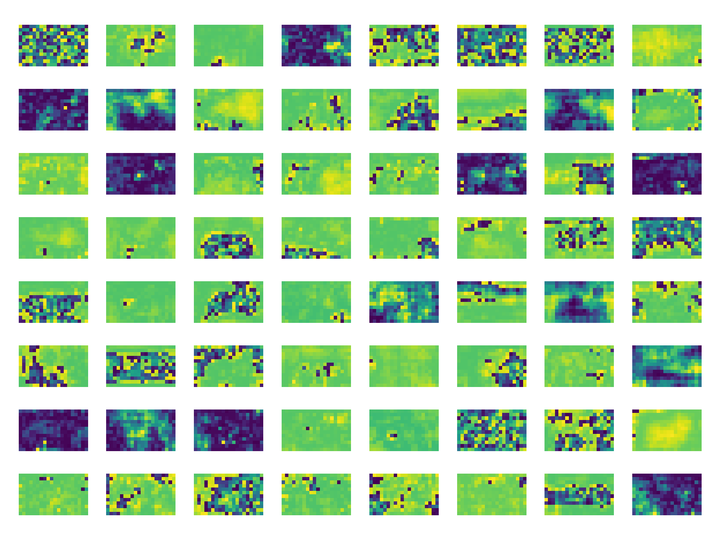### 10FPS can be used with yolov5 on the Raspberry Pi with only 0.1T computing power

Excluding the first three warm-ups, the device temperature is stable above 45°, the forward reasoning framework is ncnn, and the two benchmark comparisons are recorded

``````# 第四次
loop_count = 8
powersave = 0
gpu_device = -1
cooling_down = 1
shufflev2-yolov5  min =   90.86  max =   93.53  avg =   91.56
shufflev2-yolov5-int8  min =   83.15  max =   84.17  avg =   83.65
shufflev2-yolov5-416  min =  154.51  max =  155.59  avg =  155.09
yolov4-tiny  min =  298.94  max =  302.47  avg =  300.69
nanodet_m  min =   86.19  max =  142.79  avg =   99.61
squeezenet  min =   59.89  max =   60.75  avg =   60.41
squeezenet_int8  min =   50.26  max =   51.31  avg =   50.75
mobilenet  min =   73.52  max =   74.75  avg =   74.05
mobilenet_int8  min =   40.48  max =   40.73  avg =   40.63
mobilenet_v2  min =   72.87  max =   73.95  avg =   73.31
mobilenet_v3  min =   57.90  max =   58.74  avg =   58.34
shufflenet  min =   40.67  max =   41.53  avg =   41.15
shufflenet_v2  min =   30.52  max =   31.29  avg =   30.88
mnasnet  min =   62.37  max =   62.76  avg =   62.56
proxylessnasnet  min =   62.83  max =   64.70  avg =   63.90
efficientnet_b0  min =   94.83  max =   95.86  avg =   95.35
efficientnetv2_b0  min =  103.83  max =  105.30  avg =  104.74
regnety_400m  min =   76.88  max =   78.28  avg =   77.46
blazeface  min =   13.99  max =   21.03  avg =   15.37
googlenet  min =  144.73  max =  145.86  avg =  145.19
googlenet_int8  min =  123.08  max =  124.83  avg =  123.96
resnet18  min =  181.74  max =  183.07  avg =  182.37
resnet18_int8  min =  103.28  max =  105.02  avg =  104.17
alexnet  min =  162.79  max =  164.04  avg =  163.29
vgg16  min =  867.76  max =  911.79  avg =  889.88
vgg16_int8  min =  466.74  max =  469.51  avg =  468.15
resnet50  min =  333.28  max =  338.97  avg =  335.71
resnet50_int8  min =  239.71  max =  243.73  avg =  242.54
squeezenet_ssd  min =  179.55  max =  181.33  avg =  180.74
squeezenet_ssd_int8  min =  131.71  max =  133.34  avg =  132.54
mobilenet_ssd  min =  151.74  max =  152.67  avg =  152.32
mobilenet_ssd_int8  min =   85.51  max =   86.19  avg =   85.77
mobilenet_yolo  min =  327.67  max =  332.85  avg =  330.36
mobilenetv2_yolov3  min =  221.17  max =  224.84  avg =  222.60

# 第八次
loop_count = 8
powersave = 0
gpu_device = -1
cooling_down = 1
nanodet_m  min =   84.03  max =   87.68  avg =   86.32
nanodet_m-416  min =  143.89  max =  145.06  avg =  144.67
shufflev2-yolov5  min =   84.30  max =   86.34  avg =   85.79
shufflev2-yolov5-int8  min =   80.98  max =   82.80  avg =   81.25
shufflev2-yolov5-416  min =  142.75  max =  146.10  avg =  144.34
yolov4-tiny  min =  276.09  max =  289.83  avg =  285.99
nanodet_m  min =   81.15  max =   81.71  avg =   81.33
squeezenet  min =   59.37  max =   61.19  avg =   60.35
squeezenet_int8  min =   49.30  max =   49.66  avg =   49.43
mobilenet  min =   72.40  max =   74.13  avg =   73.37
mobilenet_int8  min =   39.92  max =   40.23  avg =   40.07
mobilenet_v2  min =   71.57  max =   73.07  avg =   72.29
mobilenet_v3  min =   54.75  max =   56.00  avg =   55.40
shufflenet  min =   40.07  max =   41.13  avg =   40.58
shufflenet_v2  min =   29.39  max =   30.25  avg =   29.86
mnasnet  min =   59.54  max =   60.18  avg =   59.96
proxylessnasnet  min =   61.06  max =   62.63  avg =   61.75
efficientnet_b0  min =   91.86  max =   95.01  avg =   92.84
efficientnetv2_b0  min =  101.03  max =  102.61  avg =  101.71
regnety_400m  min =   76.75  max =   78.58  avg =   77.60
blazeface  min =   13.18  max =   14.67  avg =   13.79
googlenet  min =  136.56  max =  138.05  avg =  137.14
googlenet_int8  min =  118.30  max =  120.17  avg =  119.23
resnet18  min =  164.78  max =  166.80  avg =  165.70
resnet18_int8  min =   98.58  max =   99.23  avg =   98.96
alexnet  min =  155.06  max =  156.28  avg =  155.56
vgg16  min =  817.64  max =  832.21  avg =  827.37
vgg16_int8  min =  457.04  max =  465.19  avg =  460.64
resnet50  min =  318.57  max =  323.19  avg =  320.06
resnet50_int8  min =  237.46  max =  238.73  avg =  238.06
squeezenet_ssd  min =  171.61  max =  173.21  avg =  172.10
squeezenet_ssd_int8  min =  128.01  max =  129.58  avg =  128.84
mobilenet_ssd  min =  145.60  max =  149.44  avg =  147.39
mobilenet_ssd_int8  min =   82.86  max =   83.59  avg =   83.22
mobilenet_yolo  min =  311.95  max =  374.33  avg =  330.15
mobilenetv2_yolov3  min =  211.89  max =  286.28  avg =  228.01
``````

### NCNN_Android_demo

This is a Redmi phone, the processor is Snapdragon 730G, and shufflev2-yolov5 is used for detection. The performance is as follows: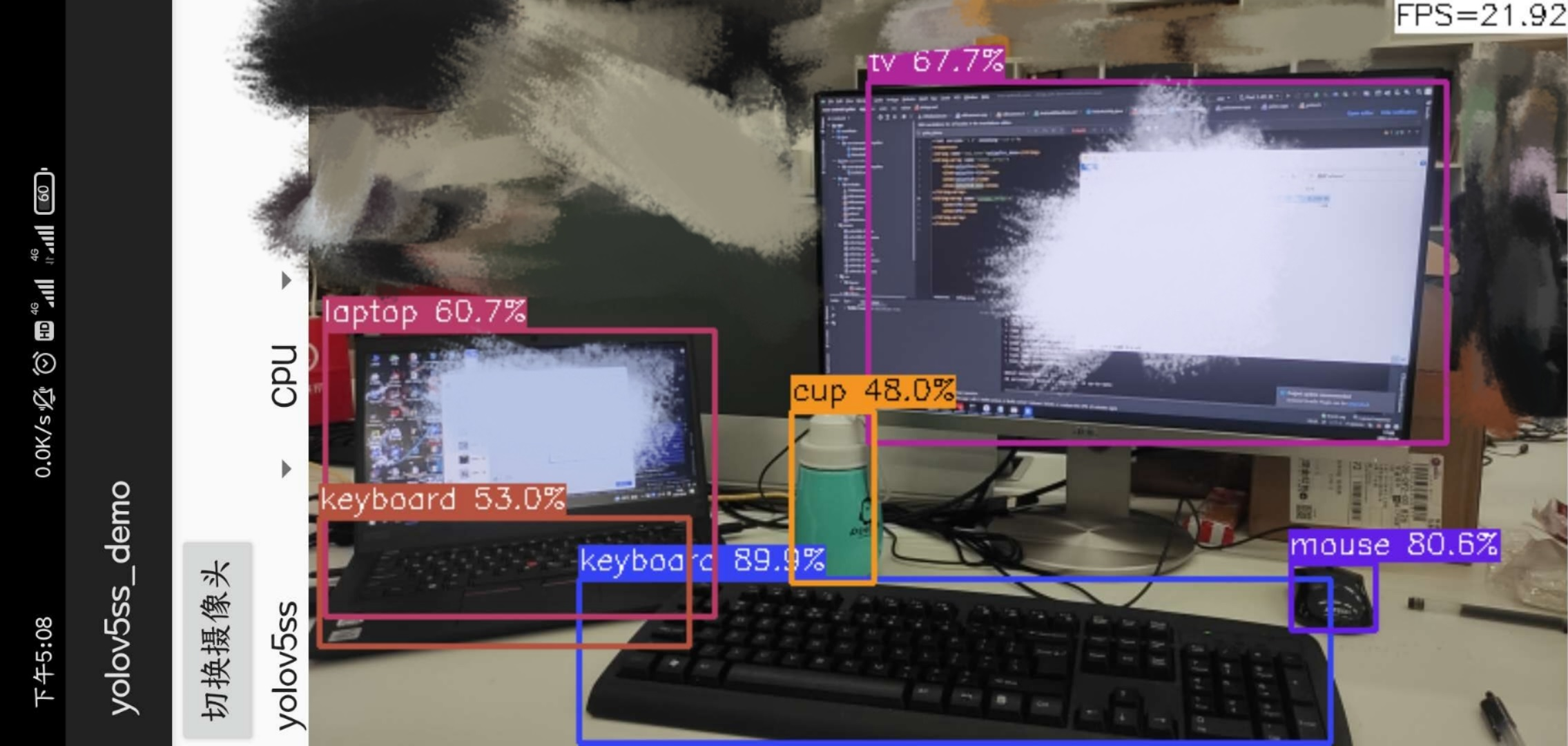This is the quantized int8 model: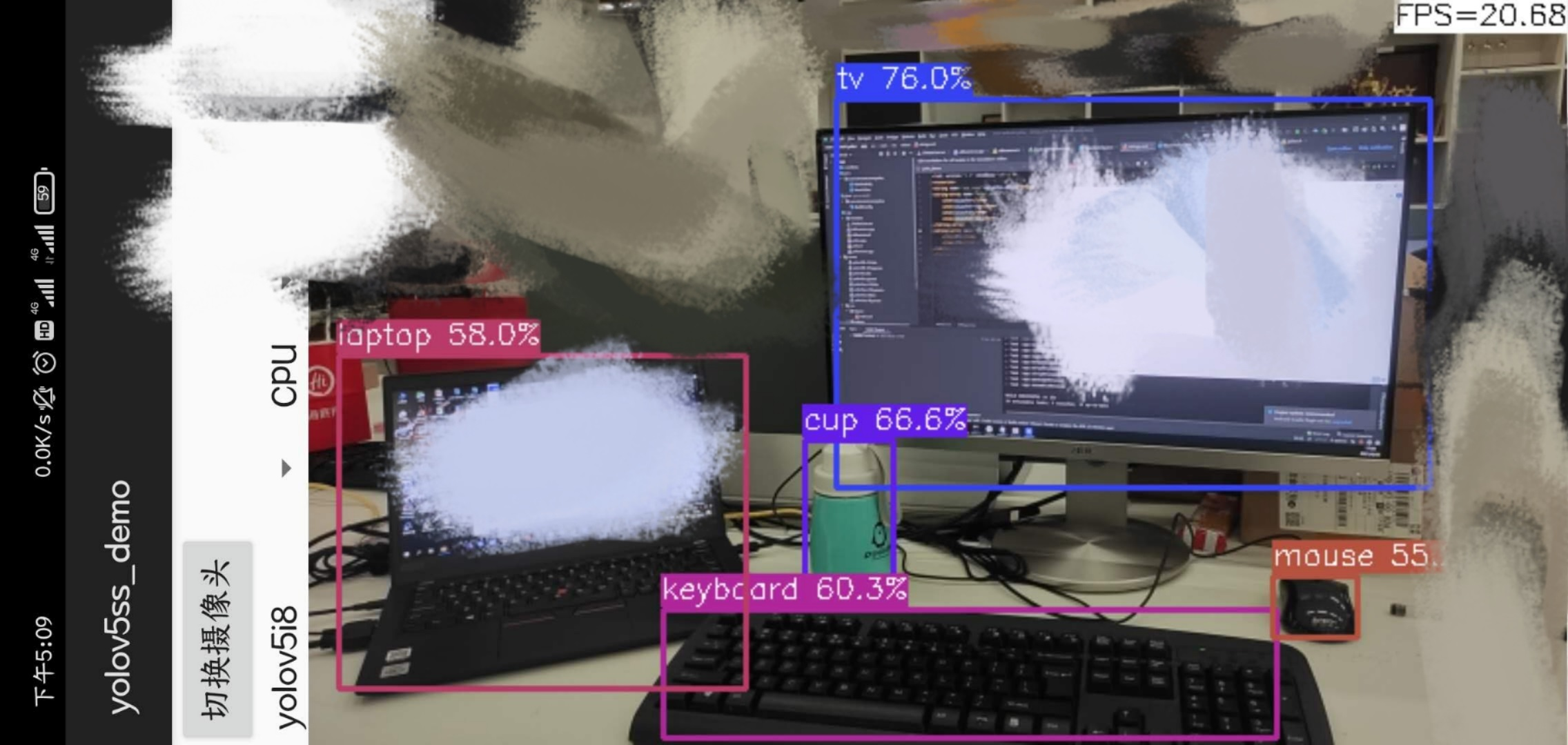Outdoor scene example: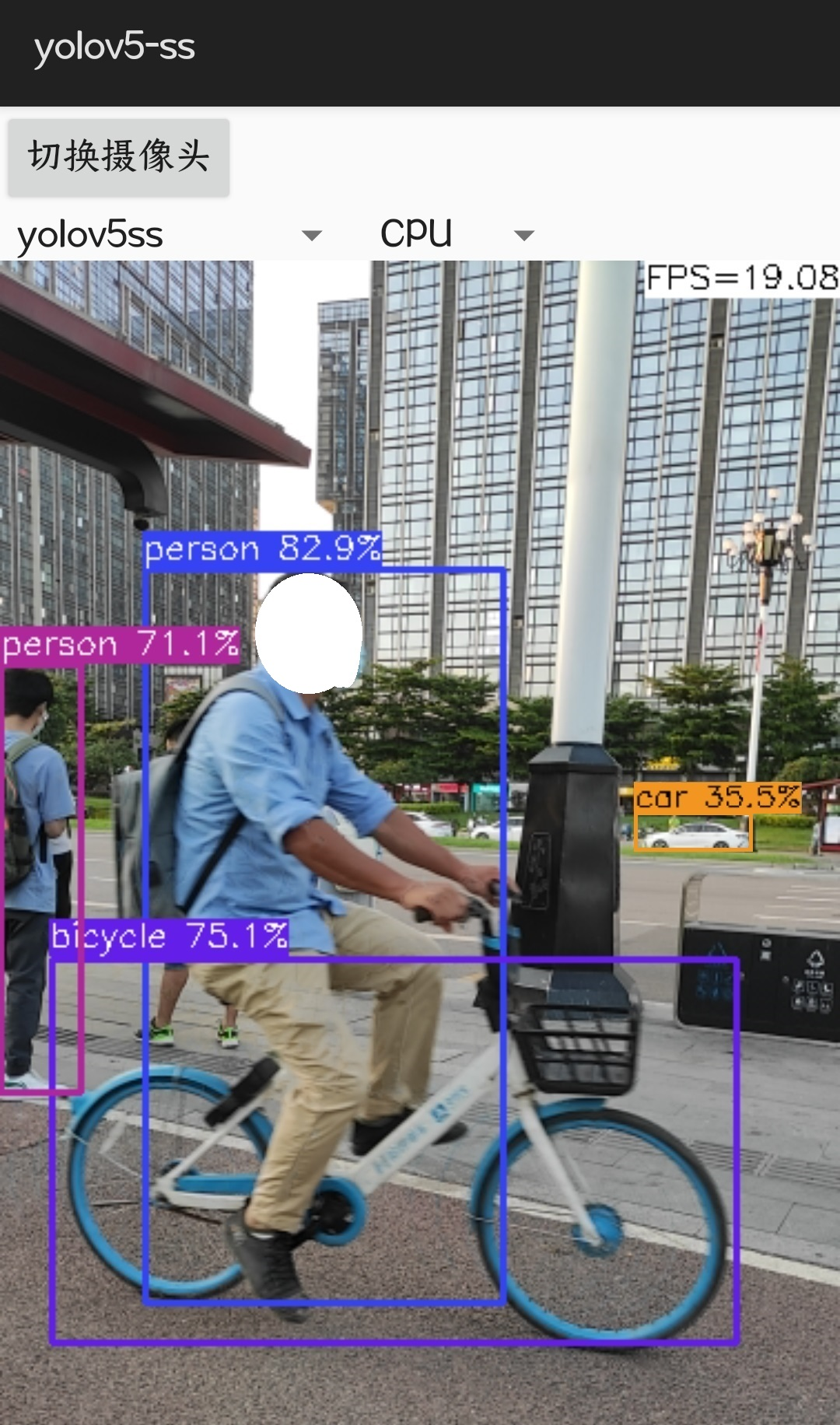### More detailed explanation